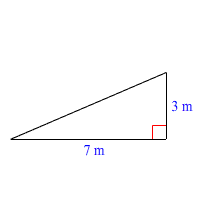# Accuracy and Error

Some math problems require an exact answer, while for others, an approximate answer is good enough.

When a problem involves measurement of a real-world quantity, there is always some level of approximation happening. For example, consider two different problems involving measures of time. If the problem involves sprinters running the $100$ -meter dash, you will need to use precise measurements: hundredths of a second or better. If the problem involves people’s ages, it’s usually enough to approximate to the nearest year.

Sometimes when a problem involves square roots or other irrational numbers like pi , or even a complicated fraction, it’s useful to use decimal approximations—at least at the end, when you’re reporting your answer.

Example 1:

Deanna is making a wire fence for her garden in the shape below. Find the length of the hypotenuse of the triangle in meters.Using the Pythagorean Theorem, we get:

$\begin{array}{l}{x}^{2}={3}^{2}+{7}^{2}\\ {x}^{2}=9+49\\ x=\sqrt{56}\end{array}$

So the hypotenuse is $\sqrt{56}$ meters long. That’s the exact answer. But having the answer in this format isn’t very useful if we’re trying to build something. How do you cut a piece of wire $\sqrt{56}$ m long?

A good calculator will tell you the value of $\sqrt{56}$ correct to $30$ decimal places:

$\sqrt{56}\approx 7.483314773547882771167497464633$

Even this is an approximation. But it’s a much better approximation that you need. In this case, rounding the value to the nearest centimeter (hundredth of a meter) is probably enough.

$\sqrt{56}=7.48$

The accuracy of a measurement or approximation is the degree of closeness to the exact value. The error is the difference between the approximation and the exact value.

When you’re working on multi-step problems, you have to be careful with approximations. Sometimes, an error that is acceptable at one step can get multiplied into a larger error by the end.

Example 2:

A plastic disk is the shape of a circle exactly $11$ inches in diameter. Find the combined area of $10,000$ such disks.

Suppose you use $3.14$ as an approximation for $\pi$ . Using the formula for the area of a circle, we get the area of one disk as:

$\begin{array}{l}A=\pi {r}^{2}\\ =\pi \cdot {11}^{2}\\ =121\pi \\ \approx 121\left(3.14\right)\\ =379.94\end{array}$

Multiply this value by $10,000$ to get the combined area of $10,000$ disks.

$379.94×10,000=3,799,400$

This gives an answer of $3,799,400$ square inches. But wait!

See what happens when we use a more accurate value for $\pi$ like $3.1416$ :

$\begin{array}{l}A=121\pi \\ \approx 121×3.1416\\ =380.1336\end{array}$

Multiply this by $10,000$ to get the combined area:

$380.1336×10,000=3,801,336$

This is almost $2000$ square inches more than our previous estimate!